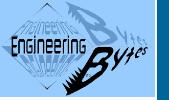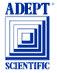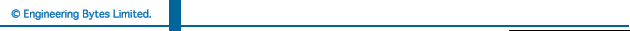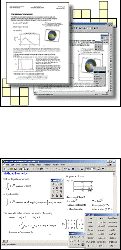Software for Civil, Structural & Geotechnic Engineers, Architects, Surveyors.Mathcad The ultimate desktop productivity tool for scientists and engineersThe Mathcad product family allows you to calculate, graph, and communicate technical ideas in an unified, easy-to-use, visual format.* Calculate, model and visualise your technical ideas* Document your calculations using familiar mathematical notation* Integrate data across software and systems* Distribute documents and collaborate across networks* Standardise across your entire organisation: Mathcad can be used by designers, technicians and managers.Use Mathcad to perform numeric calculations or to find symbolic solutions. It automatically tracks and converts units and operates on scalars, vectors, and matrices. Here is an overview of Mathcad's computational capabilities: * Numeric operators perform summations, products, derivatives, integrals and Boolean operations. * Numeric functions apply trigonometric, exponential, hyperbolic and other functions and transforms. * Symbolics simplify, differentiate, integrate, and transform expressions algebraically. Mathcad's patented live symbolics technology automatically recalculates algebraic solutions. * Vectors and Matrices manipulate arrays and perform various linear algebra operations, such as finding eigenvalues and eigenvectors, and looking up values in arrays.* Statistics and Data Analysis generate random numbers or histograms, fit data to built-in and general functions, interpolate data, and build probability distribution models. * Differential Equation Solvers support ordinary differential equations, systems of differential equations, and boundary value problems both at the command line and in solve blocks that use natural notation to specify the DiffEQs and constraints. * Variables and Units handle real, imaginary, and complex numbers with or without associated units. * High-performance calculation engine provides speed and sophisticated memory management to help you find solutions faster.